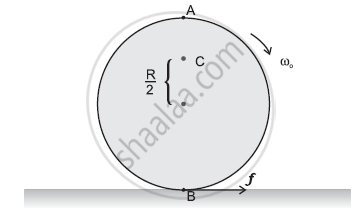CBSE (Science) Class 11CBSE
Share

Explain Why Friction is Necessary to Make the Disc and Give the Direction of Frictional Force at B, and the Sense of Frictional Torque, before Perfect Rolling Begins. and What is the Force of Friction After Perfect Rolling Begins - CBSE (Science) Class 11 - Physics

ConceptTorque and Angular Momentum

Question

Explain why friction is necessary to make the disc in Figure roll in the direction indicated

(a) Give the direction of frictional force at B, and the sense of frictional torque, before perfect rolling begins.

(b) What is the force of friction after perfect rolling begins?Solution 1

A torque is required to roll the given disc. As per the definition of torque, the rotating force should be tangential to the disc. Since the frictional force at point B is along the tangential force at point A, a frictional force is required for making the disc roll.

(a) Force of friction acts opposite to the direction of velocity at point B. The direction of linear velocity at point B is tangentially leftward. Hence, frictional force will act tangentially rightward. The sense of frictional torque before the start of perfect rolling is perpendicular to the plane of the disc in the outward direction.

(b) Since frictional force acts opposite to the direction of velocity at point B, perfect rolling will begin when the velocity at that point becomes equal to zero. This will make the frictional force acting on the disc zero.

Solution 2

To roll a disc, we require a torque, which can be provided only by a tangential force. As a force of friction is the only tangential force, in this case, it is necessary.

a)As frictional force at B opposes the velocity of point B, which is to the left, the frictional force must be to the right. The sense of frictional torque will be perpendicular to the plane of the disc and outwards.

(b)As frictional force at B decreases the velocity of the point of contact B with the surface, the perfect rolling begins only when a velocity of point B becomes zero. Also, force of friction would become zero at this stage.

Is there an error in this question or solution?

APPEARS IN

NCERT Solution for Physics Textbook for Class 11 (2018 to Current)
Chapter 7: System of Particles and Rotational Motion
Q: 29 | Page no. 181

Video TutorialsVIEW ALL 

Solution Explain Why Friction is Necessary to Make the Disc and Give the Direction of Frictional Force at B, and the Sense of Frictional Torque, before Perfect Rolling Begins. and What is the Force of Friction After Perfect Rolling Begins Concept: Torque and Angular Momentum.
S